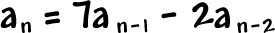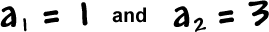Let's try another one like this.

Let's build the sequence whose nth term is given byandHere we go!

let n = 2: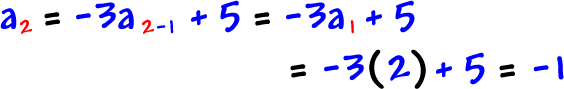let n = 3: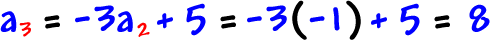let n = 4:let n = 5:So, the sequence is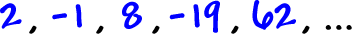*When you build a sequence, finding the first five terms will usually do the job.